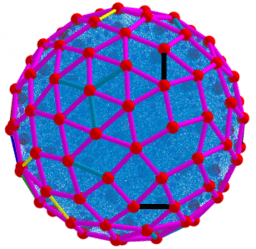# ₀Constructing polyhedron, triangulation, 3D Visualizing

Construction of a polyhedron surface based on direct calculation of the maximum sum of distances The peculiarity of this applets is that 1. for a given number of n particles on the sphere, their extreme distribution for Convex Polyhedra is found. The maximum of the sum of distances is found directly: maximization using the Maximize[]command and sliders. 2. Triangulation of polyhedral surfaces: this applets sorts and finds vertices, faces, and surface segments of a polyhedron and its dual image. 3. Visualization of polyhedral surfaces: the elements of the polyhedron and its dual image are directly translated into their 3d form. *From Book: Extended definitions of point location estimates https://www.geogebra.org/m/hhmfbvde From: List of My Public Books on GeoGebra Topics: Constructing polyhedra -https://www.geogebra.org/m/eabstecp